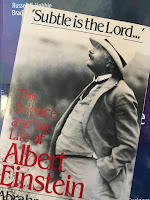## Friday, July 1, 2016

### The Wien Exponential Law

In Section 14.8 of Intermediate Physics for Medicine and Biology, Russ Hobbie and I discuss blackbody radiation. Our analysis is similar to that in many modern physics textbooks. We introduce Planck’s law for Wλ(λ,T) dλ, the spectrum of power per unit area emitted by a completely black surface at temperature T and wavelength λ
where c is the speed of light, h is Planck’s constant, and kB is Boltzmann’s constant. We then 1) express this function in terms of frequency ν instead of wavelength λ, 2) integrate over all wavelengths to derive the Stefan-Boltzmann law, and 3) show that the wavelength of peak emission decreases with temperature, often known as the Wien displacement law.

Russ and I like to provide homework problems that reinforce the concepts in the text. Ideally, the problem requires the reader to repeat many of the same steps carried out in the book, but for a slightly different case or in a somewhat different context. Below I present such a homework problem for blackbody radiation. It is based on an approximation to Planck’s law at short wavelengths derived by Wilhelm Wien.
Problem 25 ½. Consider the limit of Planck’s law, Eq. 14.33, when hc/λ is much greater than kBT, an approximation known as the Wien exponential law.
(a) Derive the mathematical form of Wλ(λ,T) in this limit.
(b) Convert Wien’s law from a function of wavelength to a function of frequency, and determine the mathematical form of Wν(ν,T).
(c) Integrate Wν(ν,T) over all frequencies to obtain the total power emitted per unit area. Compare this result to the Stefan-Boltzmann law (Eq. 14.34). Derive an expression for the Stefan-Boltzmann constant in terms of other fundamental constants.
(d) Determine the frequency νmax corresponding to the peak in Wν(ν,T). Compare νmax/T to the value obtained from Planck’s law.Subtle is the Lord, by Abraham Pais.
The Wien exponential law predated Planck’s law by several years. In his landmark biography ‘Subtle is the Lord…’: The Science and the Life of Albert Einstein, Abraham Pais discusses 19th century attempts to describe blackbody radiation theoretically.
Meanwhile,proposals for the correct form of [Wλ(λ,T)] had begun to appear as early as the 1860s. All these guesses may be forgotten except one, Wien’s exponential law, proposed in 1896…

Experimental techniques had sufficiently advanced by then to put this formula to the test. This was done by Friedrich Paschen from Hannover, whose measurements (very good ones) were made in the near infrared, λ = 1-8 μm (and T = 400 -1600 K). He published his data in January 1897. His conclusion: “It would seem very difficult to find another function…that represents the data with as few constants.” For a brief period, it appeared that Wien’s law was the final answer. But then, in the year 1900, this conclusion turned out to be premature…
And the rest, as they say, is history.